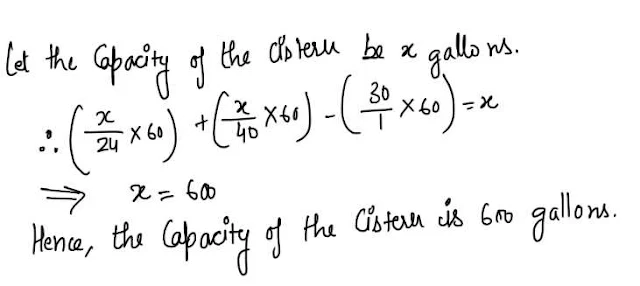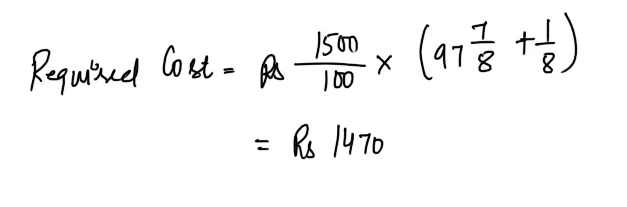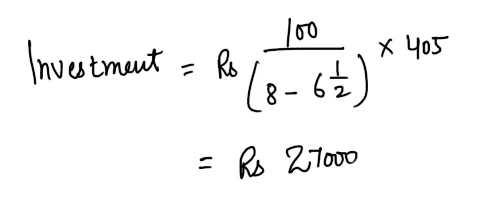Gurpurab Offer - Use Code GURPURAB2023

# Quant Quiz for IBPS PO Pre Part - 14#### Ques 1.

What is the approximate amount at compound interest of rs 5000 in 3 years at 4% p.a. ?
(a) Rs 5624
(b) Rs 5684
(c) Rs 5864
(d) None of these
Ans 1.#### Ques 2.

The simple interest on Rs 400 for 5 years together with that on Rs 600 for 4 years , the rate being same in the both the cases came to Rs 396.Rate % p.a. equals.
(a) 9
(b) 6 1/4
(c) 7 1/2
(d) 8 1/3
Ans 2.#### Ques 3.

A grocer sells 100kg of gur partly at 10% profit and partly at 20% profit, gaining 12% on his outlay. How much does he sell at 10% profit ?
(a) 88 kg
(b) 75 kg
(c) 80 kg
(d) None of these
Ans 3.#### Ques 4.

A and B can do piece of work in 60 days while B and C do the same work in days and C and A in 40 days. They all started the work together and after 20 days B and C Left. How many days more will A take to finish the work ?
(a) 24 Days
(b) 36 days
(c) 48 Days
(d) 60 days
Ans 4.#### Ques 5.

A and B can do a piece of work in 12 days, B and c in 15 days, and C and A in 20 days. How long would each take separately to do the same work ?
(a) 30 days, 20 days, 60 days.
(b) 20 days, 25 days, 50 days.
(c) 18 days, 36 days, 44 days
(d) None of the above
Ans 5.  A + B + C can together in one day do 1/2 ( 1/12 + 1/15 + 1/20 ) of the work.
i.e. 1/10 of the work.
A can do ( 1/10 -1/15) of the work i.e. 1/30 of the work in one day.
B can do ( 1/10 - 1/20) of the work i.e.1/20 of the work in one day.
C can do ( 1/10 - 1/12) of the work i.e., 1/60 of the work in one day.
Hence, A,B and C can Separately do the work in 30 days ,20 days and 60 days respectively.

#### Ques 6.

two pipes can fill a cistern separately in 24 minutes respectively and a waste pipe can drain off 30 gallons per minute .If all the three pipes are open, the cistern fills in one hour. What is the capacity of the cistern ?
(a) 300 gallons
(b) 450 gallons
(c) 600 gallons
(d) 750 gallons
Ans 6.#### Ques 7.

x is a whole number . If the only common factors which x and x2 have are 1 and x it follows that x is
(a) a perfect square
(b) an odd number
(c) equal to 1
(d) a prime number
Ans 7. Since x and x2 have only 1 and x as common factors it follows that x is a prime number.

#### Ques 8.

What is the cost of Rs 1500, 4 per cent stock at 97 7/8, brokerage 1/8 ?
(a) Rs 1480
(b) Rs 1466
(c) Rs 1470
(d) None of these
Ans 8.#### Ques 9.

If the annual rate of interest increases from 6 1/2% to 8%, a clerk 's annual income from an investment increases by Rs 405. What is his investment ?
(a) Rs 30000
(b) Rs 27000
(c) Rs 45000
(d) None of these
Ans 9.#### Ques 10.

Find the day on 1st January 2000 A.D.
(a) Monday
(b) Wednesday
(c) Friday
(d) Saturday
Ans 10. 1600 years have 0 odd days.
300 years have 1 odd days.
99 years have 24 leap years and 75 ordinary years and hence have ( 48 + 75) odd days i.e. 4 odd days
. . Total number of odd days = 0 + 1 + 4
= 5 odd days
Hence, 1st January , 2000 corresponds to 6 odd days and so the day would be Saturday.